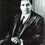# Goldbach Conjecture!

Goldbach Conjecture states that" Every even integer greater than 2 can be written as sum of two primes"

I think this might be a proof.

Let a number be 2k and let the two primes be 2n+1 and 2m+1. Therefore, 2k=2(m+n+1) k=m+n+1

Therefore, there are infinite many solutions and one of them satisfies the given equation. Goldbach conjecture is true. All who think this is wrong , please comment and make me realize my mistakeNote by Sudhir Aripirala
6 years, 5 months ago

This discussion board is a place to discuss our Daily Challenges and the math and science related to those challenges. Explanations are more than just a solution — they should explain the steps and thinking strategies that you used to obtain the solution. Comments should further the discussion of math and science.

When posting on Brilliant:

• Use the emojis to react to an explanation, whether you're congratulating a job well done , or just really confused .
• Ask specific questions about the challenge or the steps in somebody's explanation. Well-posed questions can add a lot to the discussion, but posting "I don't understand!" doesn't help anyone.
• Try to contribute something new to the discussion, whether it is an extension, generalization or other idea related to the challenge.

MarkdownAppears as
*italics* or _italics_ italics
**bold** or __bold__ bold
- bulleted- list
• bulleted
• list
1. numbered2. list
1. numbered
2. list
Note: you must add a full line of space before and after lists for them to show up correctly
paragraph 1paragraph 2

paragraph 1

paragraph 2

[example link](https://brilliant.org)example link
> This is a quote
This is a quote
    # I indented these lines
# 4 spaces, and now they show
# up as a code block.

print "hello world"
# I indented these lines
# 4 spaces, and now they show
# up as a code block.

print "hello world"
MathAppears as
Remember to wrap math in $$ ... $$ or $ ... $ to ensure proper formatting.
2 \times 3 $2 \times 3$
2^{34} $2^{34}$
a_{i-1} $a_{i-1}$
\frac{2}{3} $\frac{2}{3}$
\sqrt{2} $\sqrt{2}$
\sum_{i=1}^3 $\sum_{i=1}^3$
\sin \theta $\sin \theta$
\boxed{123} $\boxed{123}$

Sort by:

What is n and m? How do you determine them for a given k? E.g. If $k = 1000000000$, what is $n, m$?

Staff - 6 years, 5 months ago

I meant that prime is odd, so they can written in the form of 2n+1 and 2m+1. By the conjecture, let the sum of two numbers is a even number 2k=2m+2n+2 that is equal to k=m+n+1

- 6 years, 5 months ago

I understood my mistake @Calvin Lin

- 5 years, 2 months ago

That's great! Understanding your own mistakes is the first step towards discovering new facts!

Staff - 5 years, 2 months ago

You can find infinitely many solutions for some random m and n. But how do you know you can find infinitely many solutions such that 2m + 1 and 2n + 1 are both prime?

- 5 years, 11 months ago

there are infinite but only one satisfies it

- 5 years, 11 months ago

How do you know that there will be one for sure that will satisfy it? What if there are none?

- 5 years, 10 months ago